# Keep Track of Time in Excel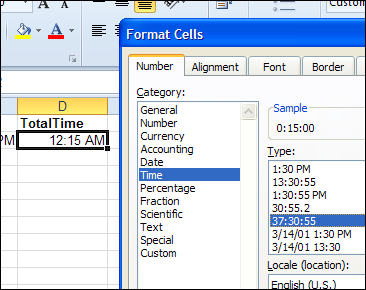If you're working on a project, you might need to keep track of time in Excel, so you can tell a client how much time you've spent on their project, and get paid for your work. Or, use the time data to see how much of your day is spent on productive tasks, and how much is spent Googling and tweeting.

### Enter the Current Time

To calculate the time spent on a task, you'll need to have a start time and an end time.

To quickly enter the current time in Excel, use the keyboard shortcut:  Ctrl + Shift + ;

• When you start a task, use the shortcut to enter the start time in one cell.
• When you finish working on a task, use the shortcut in an adjacent cell, to enter the end time.

In the screen shot below, the Task start times are entered in column B and the end times are entered in column C.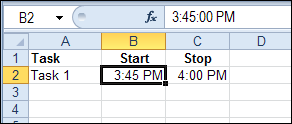### Calculate the Elapsed Time

Based on the start and end times, you can calculat the elapsed time, by subtracting the start time from the end time.

In this example, I'll calculate the time in cell D2, using this formula:

=C2-B2### Format the Elapsed Time

There is a 15 minute difference in the start and end times in this example, but the result cell is showing a time – 12:15 AM

To see the result as hours and minutes of elapsed time, change the formatting:

1. Select cell D2, where the elapsed time is calculated
2. Press Ctrl + 1 to open the Format Cells dialog box
3. On the Number tab, click the Time category
4. Click on the 37:30:55 format, then click OKThis format displays an overall total of hours, minutes and seconds. Cell D2 now shows the elapsed time of 15 minutes.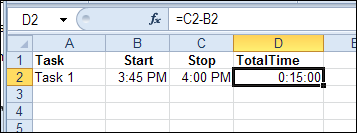### Formula Error When No End Time

The formula works well if a start time and an end time have been entered. But there is a problem if only the start time has been entered.

The result in cell D3 is a negative number, because the start time is subtracted from zero (the value of empty cell C3).

Excel displays negative dates and times as #####.To avoid this problem, add an IF function to the formula in cell D2, and copy it down to cell D3:

=IF(C2="",0,C2-B2)

Now, the formula checks cell C2, and if it is empty, the formula result is zero.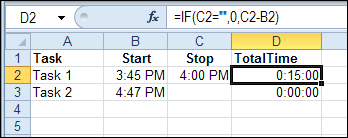### Formula Error After Midnight

If you're burning the midnight oil on client projects, you'll run into another error. In the screen shot below, the start time for Task 3 is 10:30 PM, and I worked past midnight – ending at 12:30 AM.

The formula result is a negative number, because the end time is smaller than the start time.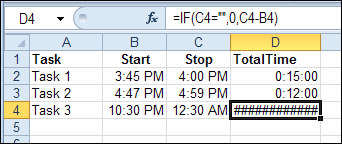To fix this problem, change the formula one more time. If the start time is greater than the end time, assume that the task ended the next day.

In that case, add 1 to the end time, which is the equivalent of adding a full day to the end time. That makes the end time greater than the start time, and the calculation will work correctly.

=IF(C2="",0,IF(B2>C2,1,0)+C2-B2)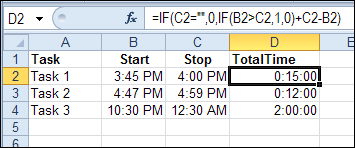To get the total time for all your tasks, use the SUM function. It should automatically format the total cell in the 37:30:55 format, but if not, you can format the cell manually.

=SUM(D2:D4)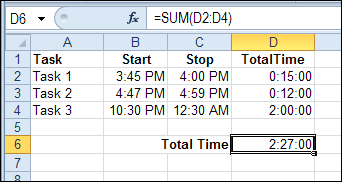________________

## 22 thoughts on “Keep Track of Time in Excel”

1. I'm actually working on a time card in Excel right now. It's pretty close to being finished. If you're interested I can post when it is completed. I'll be offering it up for free, it's helping me to learn how to do different things in Excel with VB.NET. It's been fun so far! It should come out pretty nice.

1. William says:

Hi Jon,
I'm about to start working on a time sheet, and was seeing if I could take a look at your project. Thanks!

2. Debby says:

Hi Jon,
You posted you would offer your time sheet project. I am doing something similar where I work and would love to see how you did yours. I haven't gotten into the VB part of Excel and would like to see some completed projects to back my way in. I like to see the end results and then decipher how it was accomplished. If you could email me, it would be greatly appreciated.
Thanks,
Debby

3. Jed says:

Can I have it as well?

2. Ola says:

Negative time is not possible in Excel (other than by formatting, or number to text).
This makes it especially difficult to make a bellshaped barcart chart around zero time deviation ----0++++ - from a pivottable.
I tried both formulas =IF(A10>=0;"";"-")&TEXT(ABS(A10);"tt:mm"), and formatting [>=1]D\d tt:mm;[<0]-0,00;tt:mm but Excel 2010 Chart has an error so it doesn't work.
Any other options?

3. ANAND says:

EXCELENT SUPPORT FOR EXCEL FROM THIS SITE....THANKS

4. shyam says:

Its good indeed, but any one can change the timings as per their wish.
I mean to say if we working on sharing work book, so I can make changes in others time.
so is there any way to track the time with out further corrections by any one.

5. Karen says:

I love the "after midnight" solution...have been trying to work this out for some time. But the formatting will not enable me to take the sum total and multiply it by a payrate (as in a timesheet application). Any help?

1. @Karen, multiply the total time cell by the pay rate, multiplied by 24
For example: =D6*F2*24
Then, format that cell as currency or a number with two decimal places

6. Karen says:

Beautiful! Thanks, Debra!

7. Karen says:

My timesheet is working great with one exception. When I enter the time 12:00 a.m., nothing shows up in the field...it's blank. This is the only "time figure" this happens for. The total hours worked are still calculated correctly and populate into the timesheet...but I have a blank for the end time field (I don't get the same problem with 12:00 p.m.). Any suggestions? Thanks!

1. @Karen, if your worksheet is formatted to hide zeros, then the midnight times won't show, because they are equal to zero.
To turn zeros on:
In Excel 2010, click the File tab, then click Options.
In the Advanced category, scroll down and in the Display options for this worksheet, add a check mark to "Show a zero in cells that have zero value"
Click OK to close the Options window.

8. Karen says:

Many, many thanks! I applaud your Excel expertise.

9. Kach says:

Thanks for the super quick response Debra "burning the midnight oil" 😉

10. Aziez says:

Hi
I would like to use excel to capture the time for an event. The starting time is known but the finish times will vary. Finish time needs to be in h,mm,ss. Would also like to use the space bar to capture the finish times. Any help out there? I'm not an advanced excel user.

11. Karen Rose says:

I am trying to tally a frequency count for every time someone changes a date in the date column (which represents when someone is pulled to a different assignment.) I keep getting circular references when I try to use a count function. This tally would only change as each date is updated with a new date. Any suggestions?

12. HT says:

Just found this post, this morning, because I downloaded a time tracker app, but couldn't figure out how to get it to record times on two task simultaneously. GREAT post, thank you for sharing your time and wisdom, and the many corrections. Just wanted to show how I used your formula and added to it to keep track of 7 times before totaling them.
=IF(C3="",0,IF(B3>C3, 1,0)+C3-B3)+(IF(E3="",0,IF(D3>E3, 1,0)+E3-D3))+(IF(G3="",0,IF(F3>G3, 1,0)+G3-F3))+(IF(I3="",0,IF(H3>I3, 1,0)+I3-H3))+(IF(K3="",0,IF(J3>K3, 1,0)+K3-J3))+(IF(M3="",0,IF(L3>M3, 1,0)+M3-L3))+(IF(O3="",0,IF(N3>O3, 1,0)+O3-N3))
Thought this might save someone time, if they wanted to copy and paste and then just change the cell location numbers, if needed. I would use this, for example, if you wanted to keep track of the daily times, once a week.

13. DIANA says:

I am trying to keep time for example from 8:00 am to 17:00 pm but I need to subtract 1 hr of break. How I can make the formula?

14. ark khan says:

Hi There...,
i need excel start calculating time automatically when system is locked.

15. Asha says:

Here by expect the in time result weather they come late or early and out time vise versa. for example the timings of a worker starts by 9:00:00AM and ends by 07:00:00 in this situation i want to calculate the Late hours they came or worked and OT done in the particular day

16. Rachel says:

Thank you! Still works in August of 2017 (with office 365 subscription)! What a time saver!

This site uses Akismet to reduce spam. Learn how your comment data is processed.